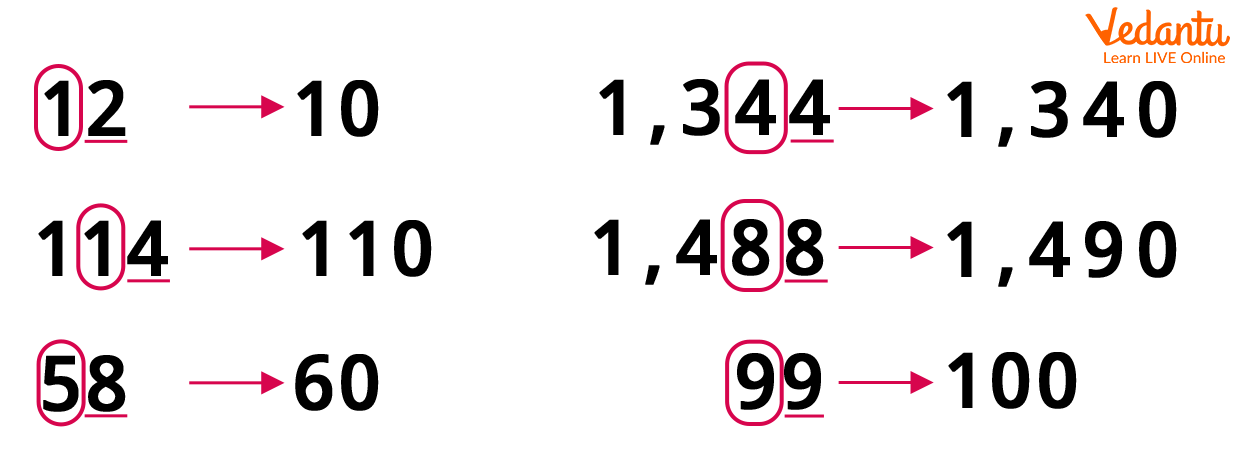Courses
Courses for Kids
Free study material
Free LIVE classes
More

# Round off to The Nearest TensLIVE
Join Vedantu’s FREE Mastercalss

## Rounding off a Number to The Nearest Tens

We have all come across instances when we failed to remember a number, date, or count simply because it was not very common or easy to recall. There are certain numbers that are easy to remember, anything in between will simply be confusing or difficult to recall.

For instance, the numbers ending with 5 or 0 are usually the ones that are remembered most easily. However, not always do we come across numbers or dates that end with 5 or 0. Therefore, to help us reach a number that ends in the desired digit, without changing the value by a lot, we use the method of rounding off to the nearest desired number. In this article, we will see how we can round a number off to the nearest tens.

## What is Round off Meaning?

Rounding a number refers to the method of conversion of a particular number into a number that is easy to deal with or remember. The rounded number may not exactly be the same as the given number, but is generally very close to the given number or an approximation.Rounding off means to estimate. Let us take an example to better understand the concept.

Example: Richard goes to the shop to buy vegetables. His total bill is ﹩98. So, he rounds off the amount to the nearest tens, which is ﹩100 and gives that to the shopkeeper. The shopkeeper easily returns the remaining ﹩2 to Richard and the deal is complete.

In the above scenario, Richard used the method of rounding off to make the calculation simpler for him and the shopkeeper. Let us now learn how this method works.

## Steps for Rounding off to The Nearest Tens

Follow the given steps to be able to round off a number to the nearest tens:

• Identify the number that needs to be rounded.

• Mark the digit in the tens column of the number.

• Check the digit in the one's column or the unit’s column, which is to the immediate right of the tens column.

• If the digit in the one’s column is 1, 2, 3, or 4, then we shall round down the number to the nearest tens.

• If the digit in the one’s column is 5 or greater, that is, 5, 6, 7, 8, or 9, then we shall round up the number to the nearest tens.

• Finally, we write the one’s place digit as 0 and change the digit in the tens place accordingly.

## Let us Understand This Better With an Example.

Consider the given number to be 87. As we have learned in the previous steps, we need to identify the unit's place or one’s place digit, which here is 7. Since 7 is greater than 5, we need to round the number ‘up’ to the nearest tens.

So, we need to change the one’s place digit to 0 and increase the tens place digit by 1, which gives us 90. Therefore, the required number obtained after rounding off 87 to the nearest tens is 90.## Conclusion

It is easy to forget numbers and counts when the one’s place digits are anything other than 0. To solve this problem, the process of rounding a number to the nearest tens is used. It helps ease the process of calculation and memorising. It is an important concept to learn and put to practical use. So do not skip this topic and try to practice these conversions as much as possible to be able to score good marks in your exams!

Last updated date: 17th Sep 2023
Total views: 138.3k
Views today: 1.38k

## FAQs on Round off to The Nearest Tens

1. If your height is 146 cm, what is your height rounded off to the nearest tens?

If the given height is 146 cm, then see the one’s place digit is 6, which is greater than 5. So, the one’s place will be 0 and the tens place will be 5, making it 150 cm. Therefore, the required rounded off height will be 150 cm.

2. If the one’s place digit is 5, should we round up or round down to the nearest tens?

In the case of the number 5 as the one’s place digit in a given number, we should always round the number up to the nearest tens.